# Algebra 1 : Decimals and Percentage

## Example Questions

### Example Question #31 : Decimals And Percentage

My minimum credit card payment is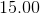dollars, and this month my total credit card bill is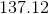dollars.  What percentage of my total bill will the minimum payment cover?  Round your answer to the nearest whole percent.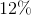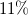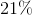Explanation:

To solve this problem, divide the amount of the minimum payment by the total billed amount.  This will yield you a decimal.  Then, to find the percentage equivalent of a decimal, simply move the decimal (if there is none, remember that there is always an implied decimal after the one's digit) to the right twice.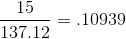or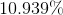Remember to round to the nearest whole percent, so the best answer is:### Example Question #32 : How To Find Percentage Equivalent To A Decimal

What is the percent equivalence of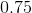?

0.75%

25%

75%

7.5%

2.5%

75%

Explanation:

This problem merely asks us to convert a decimal into a percent.

This problem may be quickly solved for by remembering that percent is a decimal that has been multiplied by. Keeping that in mind,may be multiplied byto obtain the percent equivalence.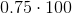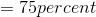### Example Question #31 : Decimals And Percentage

Which of the following is the percent equivalent of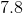?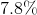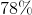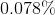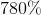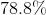Explanation:

To find the percentage equivalent of a decimal, simply move the decimal (if there is none, remember that there is always an implied decimal after the one's digit) to the right twice. In other words, multiply the decimal number by one hundred.

Thus, the answer is: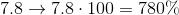### Example Question #2962 : Algebra 1

Which of the following is the percent equivalent of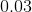?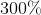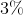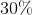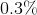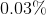Explanation:

To find the percentage equivalent of a decimal, simply move the decimal (if there is none, remember that there is always an implied decimal after the one's digit) to the right twice.

In other words multiply the decimal by one hundred.

Thus, the answer is: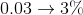or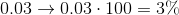### Example Question #2961 : Algebra 1

If you havedollars yet to pay on a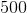dollar loan, what percentage of your loan do you still owe?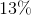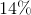Explanation:

To solve this problem, divide the amount owed by the total amount.

This will yield you a decimal.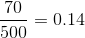Then, to find the percentage equivalent of a decimal, simply move the decimal (if there is none, remember that there is always an implied decimal after the one's digit) to the right twice. In other words, multiply the decimal by one hundred.

Thus, the answer is: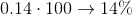### Example Question #32 : Decimals And Percentage

If you have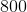dollars yet to pay on a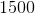dollar loan, what percentage of your loan do you still owe?

Round your answer to the nearest whole percent.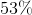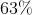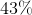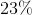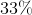Explanation:

To solve this problem, divide the amount owed by the total amount.

This will yield you a decimal.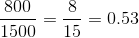Then, to find the percentage equivalent of a decimal, simply move the decimal (if there is none, remember that there is always an implied decimal after the one's digit) to the right twice. In other words, multiply the decimal by one hundred.

Thus, the answer is: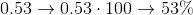### Example Question #37 : How To Find Percentage Equivalent To A Decimal

Convert the following decimal to a percent: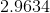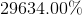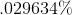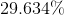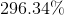Explanation:

Convert the following decimal to a percent:To convert decimals to percents, we just need to move the decimal two places to the right.

In other words, multiply the decimal by one hundred.

So,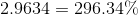And we have our answer:### Example Question #38 : How To Find Percentage Equivalent To A Decimal

Find the percent equivalent of the following decimal.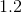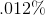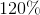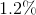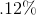Explanation:

To solve, simply convert the decimal to a percent by moving the decimal two places to the right (multiply by 100). Thus,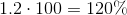### Example Question #33 : Decimals And Percentage

Write the following decimal as a percentage.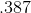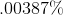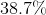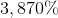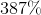Explanation:

Write the following decimal as a percentage.To write a decimal as a percent, we need to move the decimal point two spaces to the right.

SoBecomes### Example Question #31 : Decimals And Percentage

Rewrite the following decimal as a percent.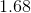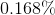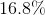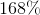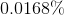Explanation:

Rewrite the following decimal as a percent.To convert between decimals and percents, we simply need to move the decimal point.

When going from a decimal to a percent, move the point two spaces to the right.

When going from a percent to a decimal, move the point two spaces to the left.

So this,Becomes### All Algebra 1 Resources